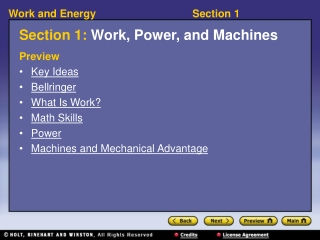DownloadDownload PresentationSection 1: Work, Power, and Machines

# Section 1: Work, Power, and Machines

Download Presentation## Section 1: Work, Power, and Machines

- - - - - - - - - - - - - - - - - - - - - - - - - - - E N D - - - - - - - - - - - - - - - - - - - - - - - - - - -
##### Presentation Transcript

1. Section 1: Work, Power, and Machines Preview • Key Ideas • Bellringer • What Is Work? • Math Skills • Power • Machines and Mechanical Advantage

2. Key Ideas • How is work calculated? • What is the relationship between work and power? • How do machines make work easier?

3. Bellringer • Which of the following is an example of work: bowling or reading? • a. A man pushes against a brick wall, which doesn’t move. Is this an example of work? b. A student carries her books to class. Is this an example of work? c. A woman raises and lowers dumbbells at the gym. Is this an example of work? d. A book falls off a table and lands on the floor. Is this an example of work?

4. What Is Work? • How is work calculated? • Work is calculated by multiplying the force by the distance over which the force is applied. • work = force x distance, orW = Fd • The force must be applied in the direction of the object’s motion.

5. What Is Work?, continued • work: the transfer of energy to an object by the application of a force that causes the object to move in the direction of the force • Work is zero when an object is not moving. • Work is measured in joules (J): 1 N • m = 1 J = 1 kg • m2/s2

6. Visual Concept: Work

7. Math Skills Work Imagine a father playing with his daughter by lifting her repeatedly in the air. How much work does he do with each lift if he lifts her 2.0 m and exerts an average force of 190 N? • List the given and unknown values. Given:force, F = 190 N distance, d = 2.0 m Unknown:work, W = ? J

8. Math Skills, continued • Write the equation for work. work = force  distance W = f  d • Insert the known values into the equation, and solve. W = 190 N 2.0 m = 380 N•m W = 380 J

9. Power • What is the relationship between work and power? • Power is the rate at which work is done, or how much work is done in a given amount of time.

10. Power, continued • power: a quantity that measures the rate at which work is done or energy is transformed • Power is measured in watts (W): 1 W = 1 J/s

11. Visual Concept: Power

12. Math Skills Power Lifting an elevator 18 m takes 100 kJ. If doing so takes 20 s, what is the average power of the elevator during the process? • List the given and unknown values. Given:work, W = 100 kJ = 1  105 J time, t = 20 s Distance is not needed. Unknown:power, P = ? W

13. Math Skills, continued 2. Write the equation for power. 3. Insert the known values into the equation, and solve.

14. Machines and Mechanical Advantage • How do machines make work easier? • Machines help do work by changing the size of an input force, the direction of the force, or both.

15. Machines and Mechanical Advantage,continued • Mechanical advantage is an important ratio. • mechanical advantage: a quantity that expresses how much a machine multiplies force or distance

16. Math Skills Mechanical Advantage Calculate the mechanical advantage of a ramp that is 5.0 m long and 1.5 m high. • List the given and unknown values. Given:input distance = 5.0 m output distance = 1.5 m Unknown:mechanical advantage = ?

17. Math Skills, continued 2. Write the equation for mechanical advantage. We need only the distance part of the full equation: 3. Insert the known values into the equation, and solve. mechanical advantage =